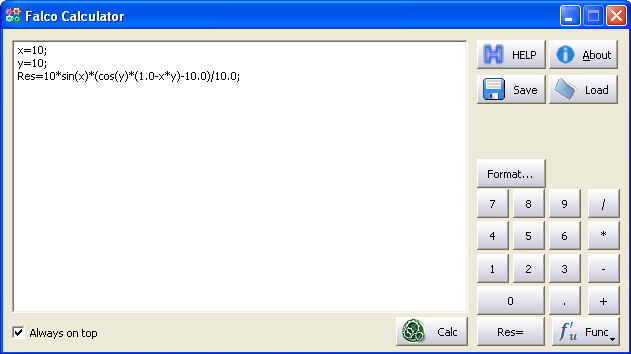• Welcome
• Interface
• Interface
• Operations
• Save
• Calculate
• Help

Welcome To The Falco Calculator Help
Falco Calculator is a useful program for education. It allows to calculate entered meanings of variables which are introduced as a formula.

Falco Calculator is usable for school or university. It economies your time and you can solve mathematical problems quickly.

Example:To calculate press "Calc" button:Example 2:

x = 0.7;

if(x != 0.7) {

Res = 1;

}

else if(x == 0.61 * 2) {

Res = 2;

}

else if(x*2 >= 1.7){

Res = 10;

}else{

Res = 3;

}

Use the help file to know more about the functionality of Falco Calculator.

Stay in touch with the most recent developments and visit the Falco Calculator Internet web site at
www.FalcoWare.com